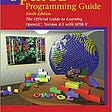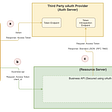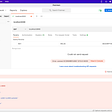# Overview

In this blog, we will enroll in creating a Java program that checks if a given matrix is a identity matrix or not. It is essential to check if the given matrix is a square matrix or not. If it is satisfied, then the condition for checking the identity matrix can be executed.

# What is identity matrix?

The formal definition of an identity matrix states that it is a square matrix in which all the elements of the principal diagonal are ones and all other elements are zeros.

The identity matrix is denoted by “I”. Its is that when a given matrix is multiplied with identity matrix, it leaves the given matrix unchanged.

# Algorithm

1. Declare and define a matrix of appropriate elements.
2. Find the number of rows and number of columns in the matrix.
3. If the number of rows is not equal to the number of columns, then print that it is not a square matrix.
4. Else, loop through each elements of the matrix and assign the flag value to be true initially.
5. Check if the diagonal elements are ones. If not, assign the flag value to be false and break the loop.
6. Check if the elements other than the diagonal elements are zeros. If not, assign the flag value to be false and break the loop.
7. If all the diagonal elements are ones and all other elements are zeros, then the flag value remains true. Print that the matrix is an identity matrix.
8. If the flag value becomes false, print that the matrix is not an identity matrix.
`if(rowLength != columnlength)     System.out.println("OOPS! The matrix is not a square matrix");else {    for(int i = 0; i < rowLength; i++) {        for(int j = 0; j < columnLength; j++) {                                                    if((i == j) && (matrix[i][j] != 1)) {                        flag = false;                break;            }            if(((i != j) && (matrix[i][j] != 0)) {                flag = false;                break;            }        }    }}`

# Source Code

Here is the complete Java program that checks if a given matrix is an identity matrix or not:

# Output

--

--

--

Love podcasts or audiobooks? Learn on the go with our new app.

## Firebase Dynamic Links- Everything You Need to Know## Serverless Server-side Rendering with Lambda Edge, CloudFront and Chrome## Ingesting Clickstream Data with Python, Kinesis, and Terraform## Starting Golang: Implementing GNU Tee## An Introspection Tale involving IBM API Connect and an external Authorization Server## TDD — nothing is white or black; all are shades of grey## How to improve quality of the automated Java tests using Pitest## Unable to hit localhost using postman